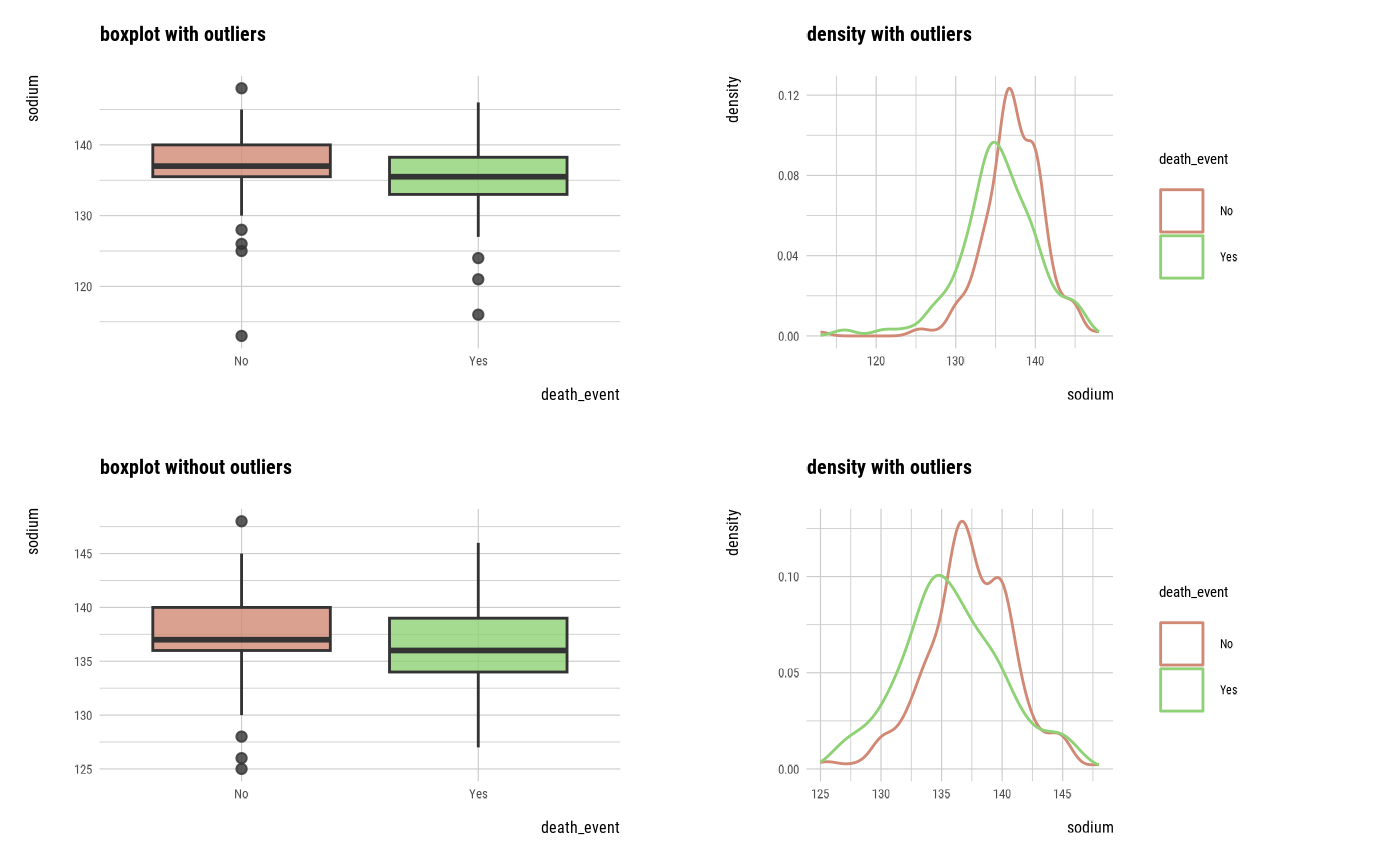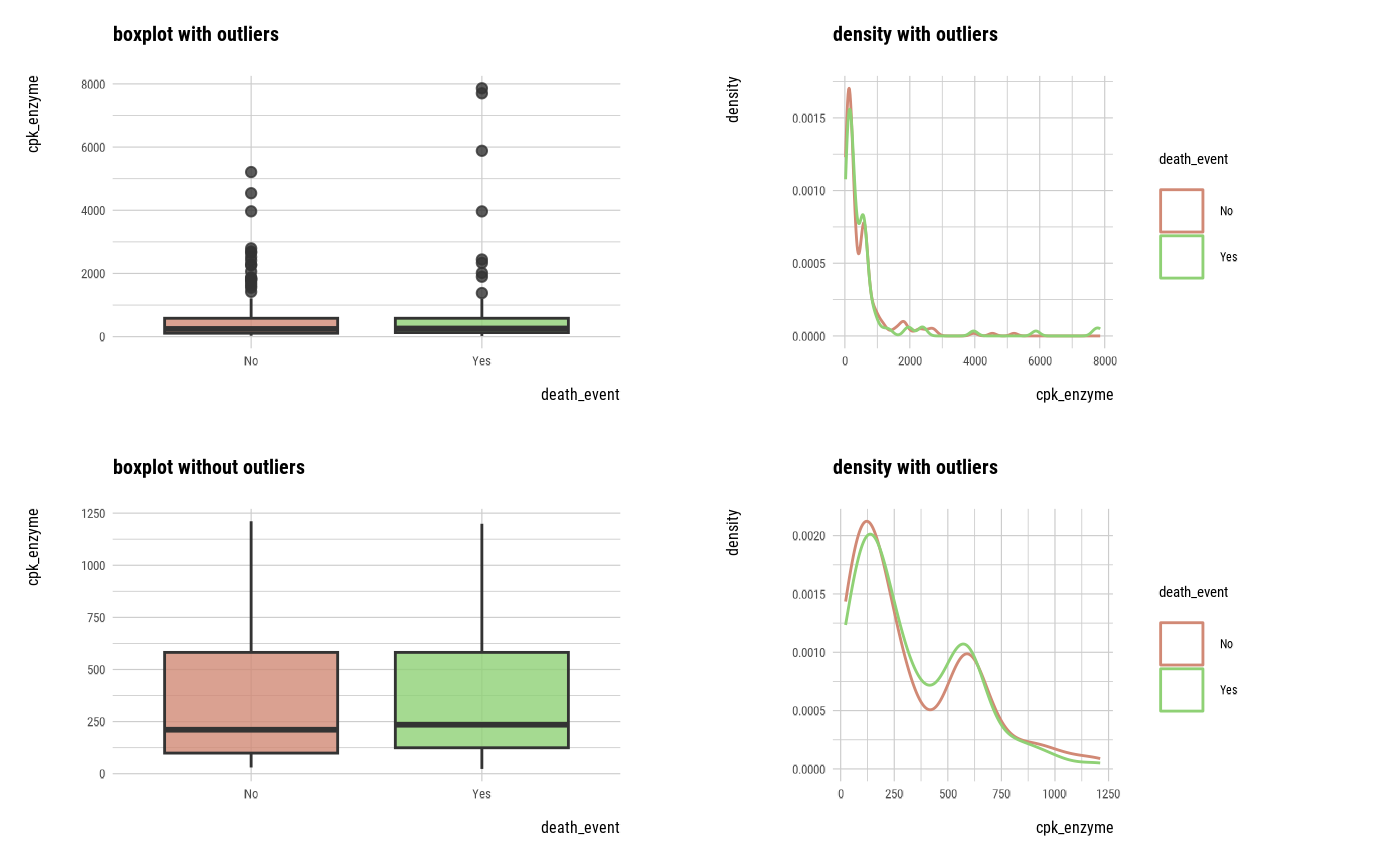The plot_outlier() visualize outlier information for diagnosing the quality of the numerical data with target_df class.

# S3 method for target_df
plot_outlier(.data, ..., typographic = TRUE, base_family = NULL)

## Arguments

.data a target_df. reference target_by. one or more unquoted expressions separated by commas. You can treat variable names like they are positions. Positive values select variables; negative values to drop variables. If the first expression is negative, plot_outlier() will automatically start with all variables. These arguments are automatically quoted and evaluated in a context where column names represent column positions. They support unquoting and splicing. logical. Whether to apply focuses on typographic elements to ggplot2 visualization. character. The name of the base font family to use for the visualization. If not specified, the font defined in dlookr is applied. (See details) The default is TRUE. if TRUE provides a base theme that focuses on typographic elements using hrbrthemes package.

## Details

The scope of the diagnosis is the provide a outlier information. Since the plot is drawn for each variable, if you specify more than one variable in the ... argument, the specified number of plots are drawn.

The base_family is selected from "Roboto Condensed", "Liberation Sans Narrow", "NanumSquare", "Noto Sans Korean". If you want to use a different font, use it after loading the Google font with import_google_font().

## Outlier diagnostic information

The plot derived from the numerical data diagnosis is as follows.

• With outliers box plot by target variable

• Without outliers box plot by target variable

• With outliers density plot by target variable

• Without outliers density plot by target variable

plot_outlier.data.frame.

## Examples

# \donttest{
# the target variable is a categorical variable
categ <- target_by(heartfailure, death_event)

plot_outlier(categ, sodium)# plot_outlier(categ, sodium, typographic = FALSE)

# death_eventing dplyr
library(dplyr)
heartfailure %>%
target_by(death_event) %>%
plot_outlier(sodium, cpk_enzyme)# death_eventing DBMS tables ----------------------------------
# connect DBMS
con_sqlite <- DBI::dbConnect(RSQLite::SQLite(), ":memory:")

# copy heartfailure to the DBMS with a table named TB_HEARTFAILURE
copy_to(con_sqlite, heartfailure, name = "TB_HEARTFAILURE", overwrite = TRUE)

# If the target variable is a categorical variable
categ <- target_by(con_sqlite %>% tbl("TB_HEARTFAILURE") , death_event)

plot_outlier(categ, sodium)# }